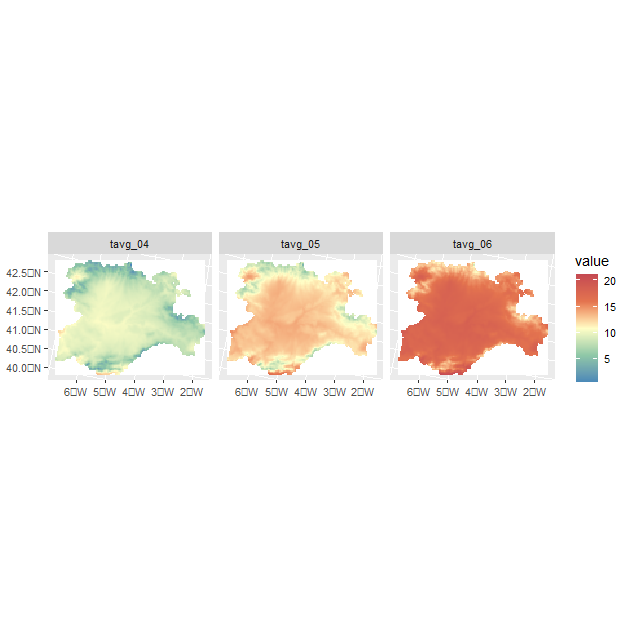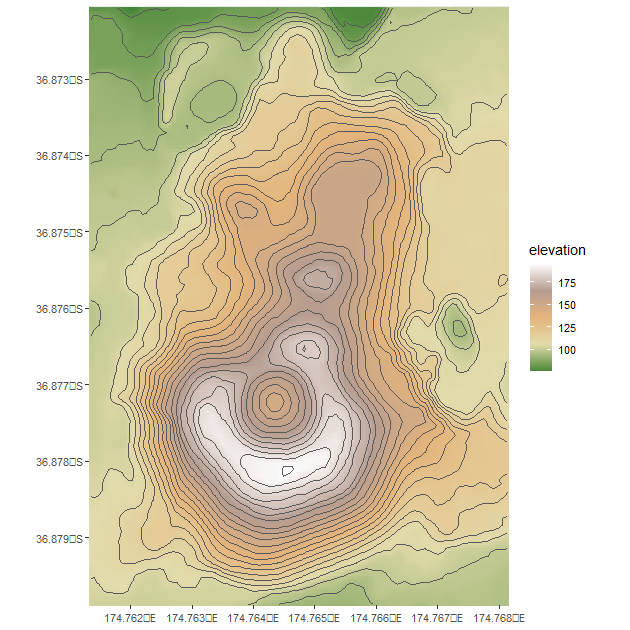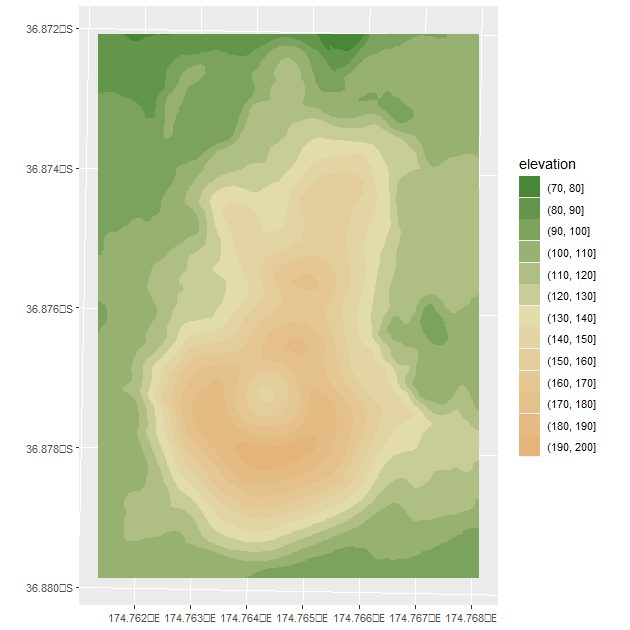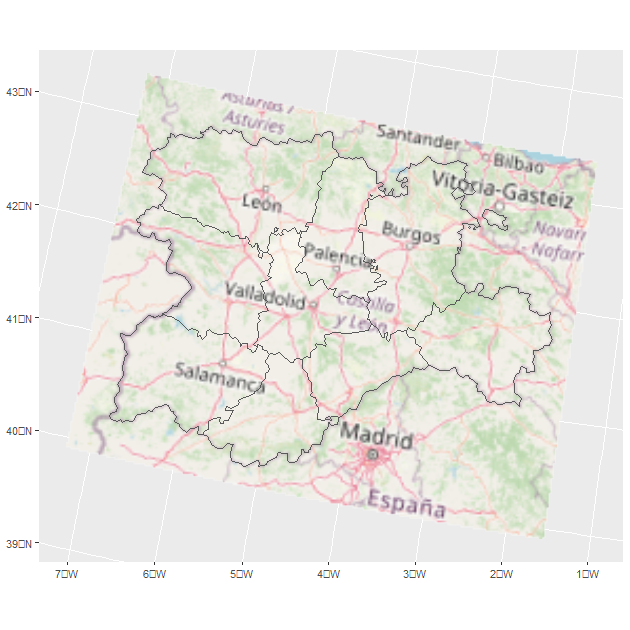# Welcome to tidyterra

## Welcome to {tidyterra}

tidyterra is a package that add common methods from the tidyverse for SpatRaster and SpatVectors objects created with the {terra} package. It also adds specific `geom_spat*()` functions for plotting these kind of objects with {ggplot2}.

### Why {tidyterra}?

Spat* objects are not like regular data frames. They are a different type of objects, implemented via the S4 object system, and have their own syntax and computation methods, implemented on the {terra} package.

By implementing tidyverse methods for these objects, and more specifically {dplyr} and {tidyr} methods, a useR can now work more easily with Spat*, just like (s)he would do with tabular data.

Note that in terms of performance, {terra} is much more optimized for working for this kind of objects, so it is recommended also to learn a bit of {terra} syntax. Each function of {tidyterra} refers (when possible) to the corresponding equivalent on {terra}.

## A note for advanced {terra} users

As previously mentioned, {tidyterra} is not optimized in terms of performance. Specially when working with `filter()` and `mutate()` methods, it can be slow.

As a rule of thumb, {tidyterra} can handle objects with less than 10.000.000 slots of information(i.e., `terra::ncell(a_rast) * terra::nlyr(a_rast) < 10e6`).

## Get started with {tidyterra}

``````library(tidyterra)
library(dplyr)
#>
#> Attaching package: 'dplyr'
#> The following objects are masked from 'package:stats':
#>
#>     filter, lag
#> The following objects are masked from 'package:base':
#>
#>     intersect, setdiff, setequal, union
library(tidyr)``````

Currently, the following methods are available:

tidyverse method SpatVector SpatRaster
`tibble::as_tibble()` ✔️ ✔️
`dplyr::filter()` ✔️ ✔️
`dplyr::mutate()` ✔️ ✔️
`dplyr::pull()` ✔️ ✔️
`dplyr::relocate()` ✔️ ✔️
`dplyr::rename()` ✔️ ✔️
`dplyr::select()` ✔️ ✔️
`dplyr::slice()` ✔️ ✔️
`dplyr::transmute()` ✔️ ✔️
`tidyr::drop_na()` ✔️ ✔️ (questioned)
`tidyr::replace_na()` ✔️ ✔️
`ggplot2::autoplot()` ✔️ ✔️
`ggplot2::fortify()` ✔️ to sf with `sf::st_as_sf()` ✔️ To tibble with coordinates

Let’s see some of them in action:

``````library(terra)
f <- system.file("extdata/cyl_temp.tif", package = "tidyterra")

temp <- rast(f)

temp
#> class       : SpatRaster
#> dimensions  : 89, 116, 3  (nrow, ncol, nlyr)
#> resolution  : 3856.617, 3856.617  (x, y)
#> extent      : 2893583, 3340950, 2019451, 2362690  (xmin, xmax, ymin, ymax)
#> coord. ref. : ETRS89-extended / LAEA Europe (EPSG:3035)
#> source      : cyl_temp.tif
#> names       :   tavg_04,   tavg_05,   tavg_06
#> min values  :  0.565614,  4.294102,  8.817221
#> max values  : 13.283829, 16.740898, 21.113781

mod <- temp %>%
select(-1) %>%
mutate(newcol = tavg_06 - tavg_05) %>%
relocate(newcol, .before = 1) %>%
replace_na(list(newcol = 3)) %>%
rename(difference = newcol)

mod
#> class       : SpatRaster
#> dimensions  : 89, 116, 3  (nrow, ncol, nlyr)
#> resolution  : 3856.617, 3856.617  (x, y)
#> extent      : 2893583, 3340950, 2019451, 2362690  (xmin, xmax, ymin, ymax)
#> coord. ref. : ETRS89-extended / LAEA Europe (EPSG:3035)
#> sources     : memory
#>               memory
#>               memory
#> names       : difference,   tavg_05,   tavg_06
#> min values  :   2.786910,  4.294102,  8.817221
#> max values  :   5.408157, 16.740898, 21.113781``````

On the previous example, we had:

• Eliminated the first layer of the raster `tavg_04`.

• Created a new layer `newcol` as the difference of the layers `tavg_05` and `tavg_06.`

• Relocated `newcol`as the first layer of the SpatRaster

• Replaced the `NA` cells on `newcol` with `3`.

• Renamed `newcol` to difference.

In all the process, the essential properties of the SpatRaster (number of cells, columns and rows, extent, resolution and coordinate reference system) have not been modified. Other methods as `filter()`, `slice()` or `drop_na()` can modify these properties, as they would do when applied to a data frame (number of rows would be modified on that case).

## Plotting with {ggplot2}

### SpatRasters

{tidyterra} provides several `geom_*` for SpatRasters. When the SpatRaster has the CRS informed (i.e. `terra::crs(a_rast) != ""`), the geom uses `ggplot2::coord_sf()`, and may be also reprojected for adjusting the coordinates to other spatial layers:

``````
library(ggplot2)

# A faceted SpatRaster

ggplot() +
geom_spatraster(data = temp) +
facet_wrap(~lyr) +
scale_fill_whitebox_c(
palette = "muted",
na.value = "white"
)````````````

# Contour lines for a specific layer

f_volcano <- system.file("extdata/volcano2.tif", package = "tidyterra")
volcano2 <- rast(f_volcano)

ggplot() +
geom_spatraster(data = volcano2) +
geom_spatraster_contour(data = volcano2, breaks = seq(80, 200, 5)) +
scale_fill_whitebox_c() +
coord_sf(expand = FALSE) +
labs(fill = "elevation")````````````

# Contour filled

ggplot() +
geom_spatraster_contour_filled(data = volcano2) +
scale_fill_whitebox_d(palette = "atlas") +
labs(fill = "elevation")``````With {tidyterra} you can also plot RGB SpatRasters to add imagery to your plots:

``````

f_v <- system.file("extdata/cyl.gpkg", package = "tidyterra")
v <- vect(f_v)

f_rgb <- system.file("extdata/cyl_tile.tif", package = "tidyterra")

r_rgb <- rast(f_rgb)

rgb_plot <- ggplot(v) +
geom_spatraster_rgb(data = r_rgb) +
geom_spatvector(fill = NA, size = 1)

rgb_plot````````````# Change CRS automatically

rgb_plot +
coord_sf(crs = 3035)``````{tidyterra} provides selected scales that are suitable for creating hypsometric and bathymetric maps:

``````
asia <- rast(system.file("extdata/asia.tif", package = "tidyterra"))

asia
#> class       : SpatRaster
#> dimensions  : 232, 432, 1  (nrow, ncol, nlyr)
#> resolution  : 22550.66, 22512.94  (x, y)
#> extent      : 7619120, 17361007, -1304745, 3918256  (xmin, xmax, ymin, ymax)
#> coord. ref. : WGS 84 / Pseudo-Mercator (EPSG:3857)
#> source      : asia.tif
#> name        : file44bc291153f2
#> min value   :        -10071.50
#> max value   :          6064.73

ggplot() +
geom_spatraster(data = asia) +
scale_fill_hypso_tint_c(
palette = "gmt_globe",
labels = scales::label_number(),
breaks = c(-10000, -5000, 0, 2500, 5000, 8000),
guide = guide_colorbar(
direction = "horizontal",
title.position = "top",
barwidth = 20
)
) +
labs(
fill = "elevation (m)",
title = "Hypsometric map of Asia"
) +
theme_minimal() +
theme(legend.position = "bottom")``````